# HBSE 8th Class Science Solutions Chapter 11 Force and Pressure

Haryana State Board HBSE 8th Class Science Solutions Chapter 11 Force and Pressure Textbook Exercise Questions and Answers.

## Haryana Board 8th Class Science Solutions Chapter 11 Force and Pressure

HBSE 8th Class Science Force and Pressure Textbook Questions and Answers

Question 1.
Give two examples each of situations in which you push or pull to change the state of motion of objects.
(i) We push the door to open it.
(ii) We pull the desk to change its position.

Question 2.
Give two examples of situations in which applied force causes a change in the shape of an object.
When we apply force on clay with our fingers, its shape gets changed, when we press balloon with our hands, its shape get changed.Question 3.
Fill in the blanks in the following statements :
(a) To draw water from a well we have to _________ at the rope.
(b) A charged body _________ an uncharged body towards it.
(c) To move a loaded trolley we have to _________ it.
(d) The north pole of a magnet _________ the north pole of another magnet.
(a) pull
(b) attracts
(c) push it
(d) repels.

Question 4.
An archer stretches her bow while taking aim at the target. She then releases the arrow, which begins to move towards the target. Based on this information fill up the gaps in the following statements using the followiong terms:
muscular, contact, non-contact, gravity, friction, shape, attraction
(a) To stretch the bow, the archer applies a force that causes a change in its _________
(b) The force applied by the archer to stretch the bow is an example of _________ force.
(c) The type offeree responsible for a change in the state of motion of the arrow is an example of a _________ force.
(d) While the arrow moves towards its target, the forces acting on it are due to _________ and that due to _________ of air.
(a) shape
(b) muscular
(c) contact
(d) gravity and friction.

Question 5.
In the following situations identify the agent exerting a force and the object on which it acts. State the effect of the force in each case.
(a) Squeezing a piece of lemon between the fingers to extract its juice.
(b) Taking out paste from a toothpaste tube.
(c) A load suspended from a spring while its other end is on a hook fixed to a wall.
(d) An athlete making a high jump to clear the bar at a certain height.
(a) Agent are fingers, object is lemon, effect of force can be observable in form of lemon juice being expelled by squeezing.

(b) Agent is hand of the person squeezing the tube, object is toothpaste tube and effect of the force can be observed’ as the paste coming out of the tube.

(c) Agent is the load suspended, object is the spring and effect can be seen in the form of elongation of spring on suspension of load.

Question 6.
A blacksmith hammers a hot piece of iron while making a tool. How does the force due to hammering affect the piece of iron?
The force due to hammering causes the change in the shape of the iron and iron can be moulded in the shape of the required tool.Question 7.
An inflated balloon was pressed against a wall after it has been rubbed with a piece of synthetic cloth. It was found that the balloon sticks to the wall. What force might be responsible for, the attraction between the balloon and the wall?
Electrostatic force.

Question 8.
Name the forces acting on a plastic bucket containing water held above ground level in your hand. Discuss why the forces acting on the bucket do not bring a change in its state of motion.
Forces acting on the plastic bucket are the muscular force and gravitational force. The forces do not bring the change in its state of motion because they are acting in opposite direction with equal magnitude. The effect of the gravitational force will pull it down if the muscular force will grow weak. The body will feel the stretch of gravitational force and will have to bend” to cancel the magnitude of gravitational force.

Question 9.
A rocket has been fired upwards to launch a satellite in its orbit. Name the two forces acting on the rocket immediately after leaving the launching pad.
Gravitational force and frictional force.

Question 10.
When we press the bulb of a dropper with its nozzle kept in water, air in the dropper is seen to escape in the form of bubbles. Once we release the pressure on the bulb, water gets filled in the dropper.
The rise of water in the dropper is due to
(a) pressure of water
(b) gravity of the earth
(c) shape of rubber bulb
(d) atmospheric pressure
(d) atmospheric pressure.

Extended Learning – Activities and Projects

Question 1.
Make a 50 cm x 50 cm bed of dry sand about 10 cm in thickness. Make sure that its top surface is levelled. Take a wooden or a plastic stool. Cut two strips of graph paper each with a width of 1 cm. Paste them vertically on any leg of the stool-one at the bottom and the other from the top. Now gently put the stool on the sand bed with its legs resting on the and Increase the size of sand bed if required. Now put a load, say a school bag full of books, on the seat of the stool. Mark the level of sand on the graph strip. This would give you the depth, if any, to which the legs of stool sink in sand. Next, turn the stool upside down so that now it rests on its seat on the sand bed. Note the depth to which the stool sinks now. Next, put the same load on the stool and note the depth to which it sinks in the sand. Compare the pressure exerted by the stool in the two situations.
For self attempt.

Question 2.
Take a tumbler and fill it with water. Cover the mouth of the tumbler with a thick card similar to that of a postcard Hold the tumbler with one hand while keeping the card pressed to its mouth with your other hand. Turn the tumbler upside down while keeping the card pressed to its mouth. Make sure that the tumbler is held vertical.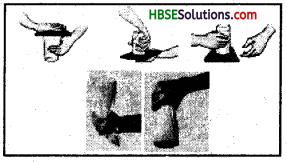Gently remove the hand pressing the card. What do you observe? Does the card get detached allowing the water to spill? With a little practice you will find that the card continues to hold water in the tumbler even after it is not supported by your hand. Also try this activity by using a piece of cloth to hold the tumbler in an upside down position.
For self attempt.

Question 3.
Take 4-5 plastic bottles of different shapes and sizes. Join them together with small pieces of glass or rubber tube as shown in Fig. Keep this arrangement on a level surface. Now pour water in any one of the bottles.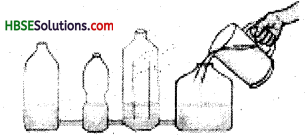Note whether the bottle in which water is poured gets filled first or all the bottle in which water is poured gets filled first or all the bottles get filled up simultaneously. Note the level of water in all the bottles from time to time. Try to explain your observations.
→ www.glenbrook.kl2 .il.us/gbssci/phys/class/ newtlauws/u212a,html
→ www.hatesville.kl2.in.us/physics/phyNet/ Mechanics/Newton2/Pressure .html
→ www.kids.earth.nasa.gov/archive/ air_pressure/
For self attempt.

Activities

Activity No. 11.1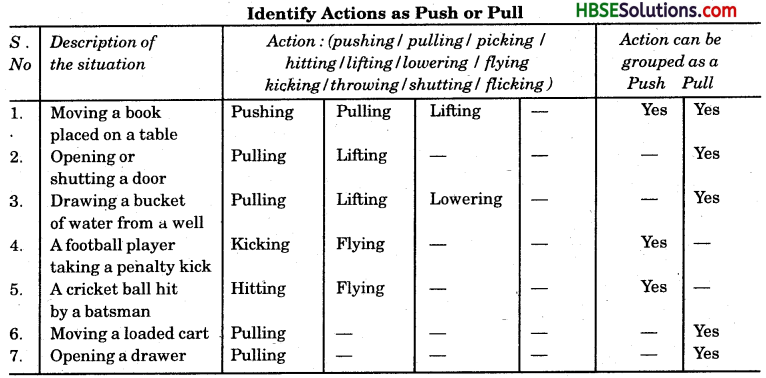Activity No. 11.5
Studying the Effect of Force on Objects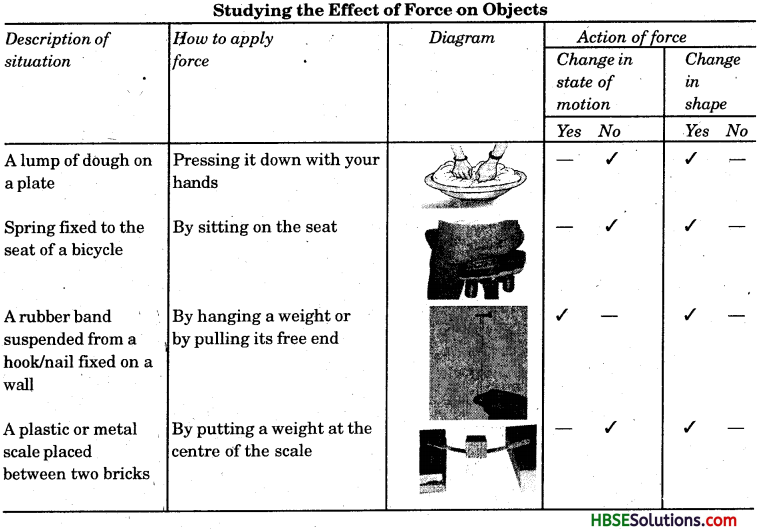Question 1.
Define force.
Any push or pull is called force.

Question 2.
When does a body move?
A body moves when force is applied.

Question 3.
What is the first requirement to put force into play?
Interaction between two objects.

Question 4.
Give any example of push.
Pushing a table to wall.Question 5.
Give an example of pull.
Pulling the door to shut.

Question 6.
When force is applied on the object in the same direction of motion, what happens?
The speed will increase.

Question 7.
What will happen when force is applied in the direction opposite to the movement of object?
The speed will decrease.

Question 8.
How is the strength of a force expressed?
In magnitude.

Question 9.
What effect does force put of state of motion?
The speed of the object may change.

Question 10.
What is the state of motion of an object?
The speed and direction of an object is called state of motion.

Question 11.
Does the force change the direction of the moving object?
Yes.

Question 12.
Does the force change the shape of the object?
Yes.

Question 13.
What is the contact force?
Force which comes in action when objects are in contact.

Question 14.
What is the muscular force?
Force resulting due to action of muscles.

Question 15.
Give examples of muscular force.
Bending, moving, kicking etc.

Question 16.
What kind of force is the muscular force?
Contact force.

Question 17.
What is friction?
Force acting equal and opposite to the moving object is friction.

Question 18.
What kind oi force is the friction?
Contact force.

Question 19.
Give example of friction force.
Stopping of a rolling ball.Question 20.
Which force stops the moving striker on a carrom board?
Friction.

Question 21.
Why does the soal of our shoes get torn away by walking?
Due to friction between soal and road.

Question 22.
What are non-contact forces?
Forces acting jrom distance without forming contact.

Question 23.
What is magnetic force?
Force exerted by a magnet is called magnetic force.

Question 24.
What happens when similar poles of magnets come in contact?
They repel each other.

Question 25.
What happens when opposite poles of magnets come in contact?
They attract each other.

Question 26.
What is the electrostatic force?
Force exerted by the charged bodies is called electrostatic force.

Question 27.
When a comb rubbed against hair is brought near the small pieces of paper, the paper attaches to the comb which force has attracted papers?
Electrostatic force.

Question 28.
What kind of forces are magnetic force and electrostatic force?
Non-contact forces.

Question 1.
How do we feel force in our daily life?
Many big or small actions make us feel the force. We have to push or pull many objects daily. A moving ball stops on its own, the ball changes the direction of its motion, when hits with a bat. We churn curd to make lassi and many other actions.

Question 2.
What is force? Define with example.
Force is the pull or push of the objects. Actions like pushing, pulling, bending, running, lifting etc. are the examples of force. Moving or stopping or changing shape and direction of motion of objects are the various actions showing force in play.

Question 3.
What happens when two people push an object from same direction?
When two people push or pull something together in same direction, the force applied by them get added and the action becomes easy.Question 4.
How can force change the state of motion?
Force can change the state of motion by changing the direction of the motion and by changing the speed of the motion. Force in the direction of the motion increases the speed and force in opposite direction decreases the speed.

Question 5.
What is the state of motion?
State of motion of an object is its speed and its direction. State erf zero motion means state of rest. An object can be in two positions one at rest or at motion. Any change in this position can be the change in the state of motion.

Question 6.
What is muscular force? Why is it a contact force?
The force exerted by the action of the muscles is called muscular force. Muscular activities like running, bending, walking etc. produce muscular force. Muscular force is a contact force because it is applied only when it is in contact with an object.

Question 7.
What is force of Motion? How does it arise?
Force of friction is force opposite and equal to the force of moving body. Force of friction arises when there is a contact between two surfaces and some movement takes place in them. Force of friction is a contact force as it only arises when two surfaces come in contact with each other.

Question 8.
Why does a moving ball gradually come to rest?
A moving bail comes to rest on its own due to force of friction. When ball moves on the ground, their surfaces come in contact to each other. The ball and the ground exert equal and opposite force on each other, which gradually brings the moving ball to a stop.

Question 9.
What is electrostatic force? What kind of a force is it?
Electrostatic force is exerted by a charged body on another charged or uncharged body. For this it is not necessary for the two bodies to be in contact with each other. This force is applicable from a distance so it is a non-contact force.Question 10.
What is pressure? What is the relation of pressure with area on which it is applied?
Force exerted on per unit area is called pressure. Pressure is related with area on which it is applied. When the area is increased the pressure exerted is less. But when the area on which pressure is exerted is decrease the pressure increases. So we conclude that pressure increases with decrease in area.

Question 1.
How will you prove that pressure of liquids decrease with increase in height ^ of its column?
For this to be proven take aplastic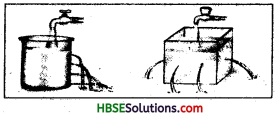container. Take a pin and make holes in the container in horizontal line from bottom to middle of the container. Now put water in the container and observe, you will see the water will come out with maximum pressure while the hole at the top will have the minimum pressure of water.

Question 2.
What changes are caused dud to application of farce on object?
Force when applied on an object cause many changes on the object. Following changes occur due to application of force:
(i) Force change the state of motion : Force can change the state of motion of the object. It can increase or decrease the speed of the moving object. It can change the direction of the moving object.

(ii) Force can change the shape of the object: Force can change the shape of the object on which it is applied. When force is applied on some object, it always have some effect on the shape of the object. The change in the object is some time visible and some time, it is pot visible, but change in shape of the object is always accompanied by application of force.

Question 3.
What are the contact forces? Explain different forces which are contact forces.
Contact forces are the forces which come in play when two objects come in contact with each other. Following forces are the contact forces :
(i) Muscular Force : The force which is exerted due to action of muscles is called muscular force. Actions like bending, running, moving etc. are examples of muscular forces. It is a contact force because it comes in play when it comes in contact of the object.

(ii) Friction: Equal and opposite force being exerted on an object when its surface comes in contact with other surface. It is also a contact force because it is exerted when two surfaces come in contact with each other Halting of a moving ball on its own is an example of force of friction.

Question 4.
What are the non-contact forces? Explain different non-contact forces.
Non-contact forces are those forces, which can be exerted from a distance without establishing a contact. Following forces are the non-contact forces :
(i) Magnetic force : Force exerted by a magnet on another magnet or magnetic substance like iron is called magnetic force. Like poles of a magnet repel each other and opposite poles of a magnet attract each other.

(ii) Electrostatic Force: Force exerted by a charged body on other charged body or uncharged body is called electrostatic force. If we rub a comb with our hair and bring it near some small bits of paper, it will attract the bits of paper towards itself. This is electrostatic force which acts without making a direct contact with other charged or uncharged object.

(iii) Force of Gravity : Earth pulls every freely falling object towards its centre. This force exerted by earth is called force of gravity. This is also a non-contact force as it acts from a distance.Force and Pressure Class 8 HBSE Notes

• Every push or pull is called force.
• Force comes into action when an object interacts with other object.
• Forces when implied in same direction add to each other, but when applied in opposite directions, they cancel each other. Equal forces applied in opposite direction results in net zero force.
• Force has magnitude and direction.
• Force can bring many changes in body on which it is applied.
• Force can change the speed of the moving body. Force in same direction of motion can increase the speed and in opposite direction can decrease the speed.
• Force can change the direction of the object in motion or at rest.
• Force can change theshapeof theobject. Force when applied on an object is always accompanied by change’in its shape.
• Two objects when come in contact with each other, they apply force on each other, this is called contact force. Muscular force, friction force etc. are examples of contact force.
• Force acting opposite to the direction of motion of a moving object is called force of friction.
• Force that acts from a distance is called a non-contact force, Magnetic force, electrostatic force and gravitational force are examples of non-contact force.
• Force exerted per unit area is called pressure.
• Liquids and gases also exert pressure in all directions.
• Pressure exerted by air is called atmospheric pressure.